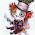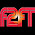## Monday, March 31, 2014

### 55 | Why Satan took Psychology | Numerology of 'School Subjects'

Below is a list of common school subjects in Pythagorean Numerology.
• Mathematics = 4+1+2+8+5+4+1+2+9+3+1 = 40
• Math = 4+1+2+8 = 15
• Science = 1+3+9+5+5+3+5 = 31
• History = 8+9+1+2+6+9+7 = 42
• Reading = 9+5+1+4+9+5+7 = 40
• Writing = 5+9+9+2+9+5+7 = 46
• Language = 3+1+5+7+3+1+7+5 = 32
• Art = 1+9+2 = 12
• Anthropology = 1+5+2+8+9+6+7+6+3+6+7+7 = 67
• Sociology = 1+6+3+9+6+3+6+7+7 = 48
• Psychology = 7+1+7+3+8+6+3+6+7+7 = 55
• Physics = 7+8+7+1+9+3+1 = 36
• Chemistry = 3+8+5+4+9+1+2+9+7 = 48
• Biology = 2+9+6+3+6+7+7 = 40
• Geology = 7+5+6+3+6+7+7 = 41
• Geography = 7+5+6+7+9+1+7+8+7 = 57
• Economics = 5+3+6+5+6+4+9+3+1 = 42
• Finance = 6+9+5+1+5+3+5 = 34
• Accounting = 1+3+3+6+3+5+2+9+5+7 = 44
• Communication = 3+6+4+4+3+5+9+3+1+2+9+6+5 = 60
• Engineering = 5+5+7+9+5+5+5+9+9+5+7 = 71
• Medicine = 4+5+4+9+3+9+5+5 = 44
Below is a list of common school subjects in Standard Numerology.
• Mathematics = 13+1+20+8+5+13+1+20+9+3+19 = 112
• Math = 13+1+20+8 = 42
• Science = 19+3+9+5+14+3+5 = 58
• History = 8+9+19+20+15+18+25 = 114
• Reading = 18+5+1+4+9+14+7 = 58
• Writing = 23+18+9+20+9+14+7 = 100
• Language = 12+1+14+7+21+1+7+5 = 68
• Art 1+18+20 = 39
• Anthropology = 1+14+20+8+18+15+16+15+12+15+7+25 = 166
• Sociology = 19+15+3+9+15+12+15+7+25 = 120
• Psychology = 16+19+25+3+8+15+12+15+7+25 = 145
• Physics 16+8+25+19+9+3+19 = 99
• Chemistry = 3+8+5+13+9+19+20+18+25 = 120
• Biology = 2+9+15+12+15+7+25 = 85
• Geology = 7+5+15+12+15+7+25 = 86
• Geography = 7+5+15+7+18+1+16+8+25 = 102
• Economics = 5+3+15+14+15+13+9+3+19 = 96
• Finance = 6+9+14+1+14+3+5 = 52
• Accounting = 1+3+3+15+21+14+20+9+14+7 = 107
• Communication = 3+15+13+13+21+14+9+3+1+20+9+15+14 = 150
• Engineering = 5+14+7+9+14+5+5+18+9+14+7 = 107
• Medicine = 13+5+4+9+3+9+14+5 = 62
I decided to breakdown the numerology of school subjects to see if there was anything curious that I would find.  Above, I have underlined and bolded what I think is interesting.  What stood out to me the most however was psychology's numerology of 55; which matches the word 'Satan'.
• Psychology = 7+1+7+3+8+6+3+6+7+7 = 55
• Satan = 19+1+20+1+14 = 55
In this world we live in, the study of psychology has not been used to help humanity.  Take a moment to think about it.  In the time we've been studying psychology, we've seen mental health decline on a global level, and we've seen a rise in mental illness; as well as a massive rise in prescription drugs used to treat that mental illness.  It also seems that the study of the brain and psychology in this same period of time has created information used by companies to lure children into become consumers at younger and younger ages instead of teaching them how to access greater capabilities hidden within their own skull.  Consumption isn't just up in children, it is up in all people, across the globe.  Further, it isn't just industry that uses psychology to manipulate people into consumption, it is governments that use "fake terrorism" campaigns and "fake news" through their FCC controlled outlets to scare people, and in turn, control their psychology, for their political purposes.

For me personally, it also reminds of ex-girlfriends who were psychology majors and thought they knew something about something because they'd earned a piece of paper from some college saying they'd accomplished something.  Most of these women want to take their piece of paper that says they know something about psychology and help people through the practice of counseling, when sadly, they can't even help themselves with their own problems.  In this regard, it is the classic case of looking outwards and trying to tend to other people's problems so that they will be distracted from their own.  Take my cousin for example, who only knows how to exit a relationship by cheating on her partners.  In a couple of years she will be a doctor, helping people with their life struggles.

That's the devil working, and that's what psychology seems to be at this day in age.  I highly doubt the numerology of the word being 55 is a mistake.  The practice of psychology seems to be tearing this world apart as much as militaries headquartered out of five sided, five floored buildings, that are opposite 555 foot tall obelisks in our nation's capitol.

When it comes to psychology, just say no.

Also, equally curious, is that 'economics' and 'Satanism' have equal numerology.
• Economics = 5+3+15+14+15+13+9+3+19 = 96
• Satanism = 19+1+20+1+14+9+19+13 = 96
Between economics and psychology, you could say the powers that be have the world under their thumb.  And to think, the brand of economics that has made the rich incredibly rich, and caused much of the world to suffer, has come out of the Chicago School of Economics, home of the 60606 area code.  If you think this is all by coincidence, you're blind.

#### 4 comments:

1.Olá,
a loucura, a neurose sempre existiu na população. Qualquer civilização que você pesquisar esteve no mesmo nível de adormecimento, independentemente de seus costumes. Esta loucura não é exclusividade do nosso tempo. A psicologia ao meu ver, apenas catalogou (superficialmente) as doenças mentais. O tratamento é péssimo, mais atrapalha do que ajuda. Na verdade induz o paciente a adquirir outra neurose. É um processo adestrador.

E psicologia da número 55, apenas na sua língua.

2.Translated: Hello, the madness, the neurosis always existed in the population. Any civilization that you search was at the same level of sleep, regardless of their customs. This madness is not exclusive of our time. The psychology in my view, only catalogd (superficially) mental illness. The treatment is very bad, more intrusive than aid. In fact induces the patient to acquire another neurosis. It is a process mahout. And the psychology of number 55, only in their own language.

3.It should be pointed out that "English" should not be minimized, as it is the most well spread language across the globe, without question. Could that change in the future? Of course. But the people in power now, they've made English as far spread as it is. This coincides with the saying, "The sun never sets on Britain".

4.Very interesting post.
I was searching your blog for gematria of the Elements of Chemistry. (I know that you posted somewhere but I cannot find it). Please help. Thank you.